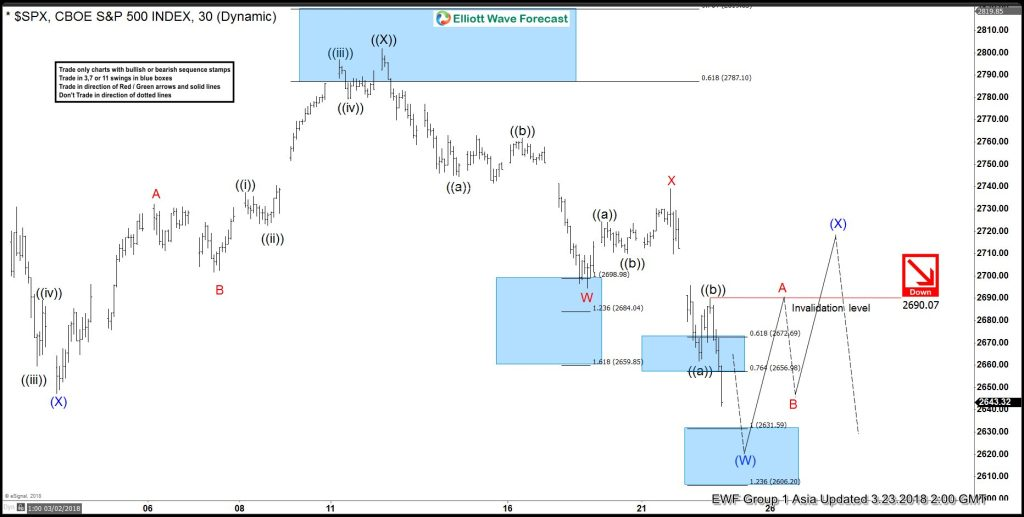# Elliott Wave Analysis: SPX Should See More Weakness

SPX rally to 2801.9 on 3.13.2018 ended Primary wave ((X)). Since then, the decline from there is unfolding as a double three Elliott Wave structure where Minor wave W ended at 2694.59 and Minor wave X ended at 2739.14. A double three is a WXY structure where the subdivision of each leg is corrective. Subdivision of Minor wave W unfolded as a zigzag Elliott Wave structure where Minute wave ((a)) ended at 2744.38, Minute wave ((b)) ended at 2761.85, and Minute wave ((c)) of W ended at 2694.59. Subdivision of Minor wave X unfolded as another zigzag where Minute wave ((a)) ended at 2724.22, Minute wave ((b)) ended at 2710.05, and Minute wave ((c)) of X ended at 2739.14.

Minor wave Y is currently in progress as a zigzag Elliott Wave structure where Minute wave ((a)) ended at 2661.78 and Minute wave ((b)) ended at 2690.07. Near term focus is on 2606.2 – 2631.59 area to complete Minute wave ((c)) of Y. The next move lower also should complete Intermediate wave (W) of higher degree and end cycle from 3.13.2018 high. Index should then bounce in Intermediate wave (X) to correct cycle from 3.13.2018 high in 3, 7, or 11 swing before the decline resumes.

#### SPX 1 Hour Elliott Wave Chart